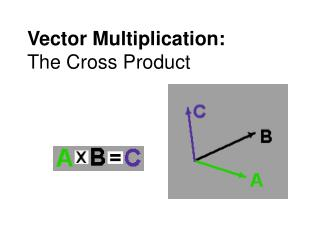DownloadDownload PresentationVector Multiplication: The Cross Product

# Vector Multiplication: The Cross Product

Download Presentation## Vector Multiplication: The Cross Product

- - - - - - - - - - - - - - - - - - - - - - - - - - - E N D - - - - - - - - - - - - - - - - - - - - - - - - - - -
##### Presentation Transcript

1. Vector Multiplication:The Cross Product

2. When two vectors are “multiplied” to form a 3rd vector, the new vector is called the cross product of the original vectors. Symbolically, you write The magnitude of the cross product is given by The direction of the cross product is perpendicular to both of the original vectors as determined by the right hand rule.

3. Recall the right hand rule

4. Unit vectors are mutually perpendicular, therefore Vector multiplication is distributive, just like ordinary multiplication, so you can find the cross product easily if the vectors are in unit vector notation. Example:

5. Practice with cross-products Consider two vectors: 1. Calculate • using the vector component method • using the cross product formula 2. Show that taking a cross product is not commutative. Cross product tutorial http://physics.syr.edu/courses/java-suite/crosspro.html

6. Application: TORQUE Consider a 10 N force applied to a rod pivoted about the left end. We can apply the force in a variety of ways, not all causing the same result: Torque is a combination of the magnitude of force applied, its direction, and distance from the axis of rotation to the point of application of the force.